# Hemmer and Stell modelThis article is a 'stub' page, it has no, or next to no, content. It is here at the moment to help form part of the structure of SklogWiki. If you add sufficient material to this article then please remove the {{Stub-general}} template from this page.

The Hemmer and Stell model intermolecular pair potential,$\Phi_{12}(r)$, is given by (Ref. 1 Eq. 2, Ref. 2 Eq. 1-3):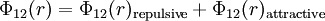$\Phi_{12}(r) = \Phi_{12}(r)_{\mathrm {repulsive}} + \Phi_{12}(r)_{\mathrm{attractive}}$

where a short range piece-wise potential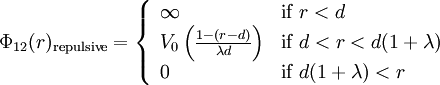$\Phi_{12}(r)_{\mathrm {repulsive}} = \left\{ \begin{array}{ll} \infty & {\rm if} \; r < d \\ V_0 \left( \frac{1- (r-d)}{\lambda d} \right)& {\rm if} \; d < r < d(1+ \lambda) \\ 0 & {\rm if} \; d( 1+ \lambda) < r \end{array} \right.$

where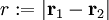$r := |\mathbf{r}_1 - \mathbf{r}_2|$,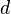$d$ is the hard core radius, along with a long-range attractive potential;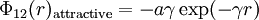$\Phi_{12}(r)_{\mathrm{attractive}} = -a\gamma \exp (-\gamma r)$

where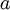$a$ is a positive constant.